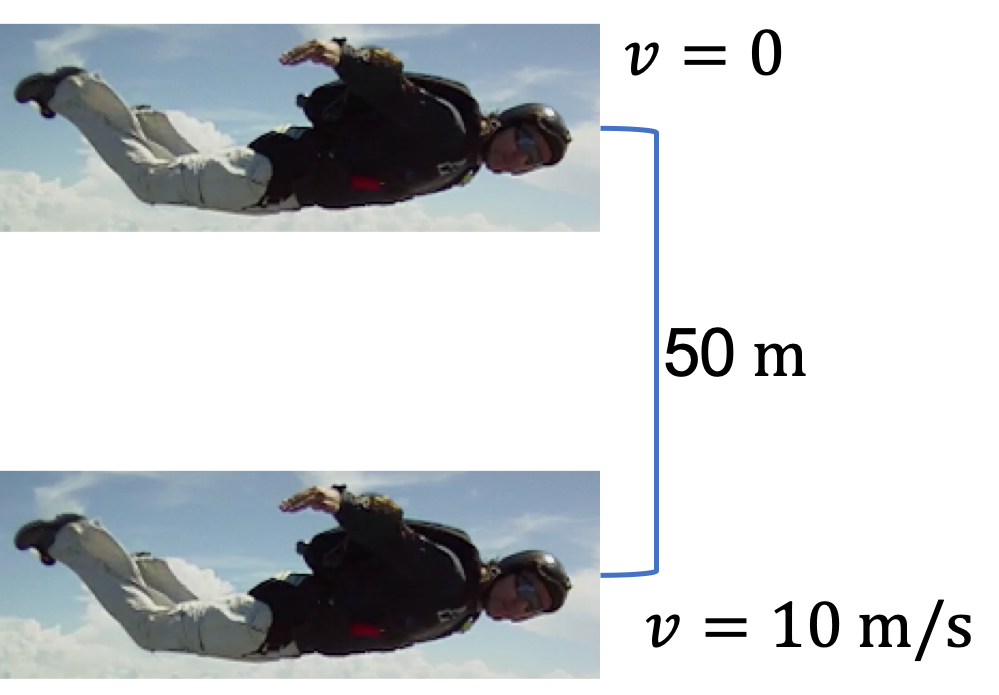## Section8.8Non-Conservation of Energy

We found above that if only conservative forces act on a system, then, energy is conserved during its motion. A conservative force was found to have work that has no path-dependence. Work by a non-conservative force, such as drag or friction, does depend on path. To handle non-conservative forces in an energy conideration, we separate net work in the work-energy theorem into contributions of conservative and non-conservative forces.

\begin{equation*} W_{if}^{\text{cons}} + W_{if}^{\text{non-cons}} = K_f - K_i, \end{equation*}

where the superscript “cons” refers to the conservative part. We know that the work by conservative forces will be equal to the difference of initial and final potential energies.

\begin{equation*} \sum U_i - \sum U_f + W_{if}^{\text{non-cons}} = K_f - K_i, \end{equation*}

where $\sum U$ stands for the sum of potential energies from all the conservative forces involved, not just one. Now, we can send the $U$'s to the right side, where we bundle them with the corresponding kinetic energy term.

\begin{equation*} W_{if}^{\text{non-cons}} = \left(K_f + \sum U_f\right) - \left(K_i+\sum U_i\right). \end{equation*}

Writing $K + \sum U$ as energy $E \text{,}$ we can rewrite this equation as

\begin{equation} W_{if}^{\text{non-cons}} = E_f - E_i.\label{eq-conservation-of-mechanical-energy}\tag{8.8.1} \end{equation}

That is, any change in energy, either increase or decrease, comes from the work by the nonconservative forces. Sometimes, we call this the general principle of conservation of energy.

A sky diver exits an air plane at almost zero speed. He falls a distance of $50\text{ m}\text{.}$ His speed at that point is $10\text{ m/s}\text{.}$ Show calculations to decide if his energy is conserved or not.Hint

Find the sign of $\Delta E\text{.}$

Not conserved

Solution

We compare energy at the later instant with the energy when the skydiver fell from the plane.

\begin{align*} \Delta E \amp = E_f - E_i = (K_f + 0) - (0 +U_i) \\ \amp = m\left( \dfrac{1}{2}\times 10^2 - 9.81\times 50 \right) = -440.5\, m\lt 0. \end{align*}

This says that the skydiver lost energy in his motion. That is, the energy of skydiver is not conserved.

A $70\text{-kg}$ skier starts from rest on top of a $40\text{ m}$ high hill. When he reaches the bottom of the hill he is going at $20\text{ m/s}\text{.}$ How much energy has he lost to air and friction?

Hint

Find $\Delta E = E_f - E_i\text{.}$

$13,500\text{ J} \text{.}$

Solution

We look at the difference in energy.

\begin{align*} \Delta E \amp = (K_f + 0) - (0 +U_i) =\dfrac{1}{2}m\,v_f^2 - m\,g\,h_i \\ \amp = \dfrac{1}{2}\times 70\times 20^2 - 70\times 9.81\times 40 = -13,500\text{ J}, \end{align*}

where I have rounded off the answer to 3 digits. The negative value of $\Delta E$ means a loss in energy. This is the work done by the nonconservative forces, which are air drag and friction.

A crate is being pushed across a rough floor surface. If no force is applied on the crate, the crate will slow down and come to a stop. The friction is such that if the crate of mass $50 \text{ kg}$ moving at speed $8 \text{ m/s}$ comes to rest in $10 \text{ sec}$ at constant deceleration. You need to continue to push on the crate if you want the motion to continue at constant speed $8\text{ m/s}\text{.}$ What would be the work done by your force in one second, also called power of the force?

Hint

In one second, the displacement $d = v\text{.}$

$320 \text{ J/s}\text{.}$
We need to supply the energy at the rate equal to the rate at which energy is removed by this friction force. The distance traveled in $1\text{ sec}$ will be equal to $v\text{.}$ Therefore, work per second, i.e., power $P\text{,}$ will be